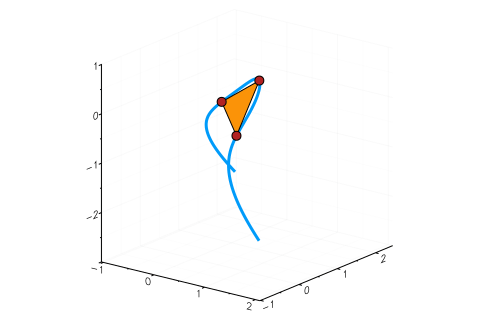# Tritangents

Using HomotopyContinuation.jl for computing tritangents of sextic curves

A complex sextic curve in $\mathbb{C}^3$ is the intersection of a cubic surface $\mathcal{C}\subset \mathbb{C}^3$ with a quadric $\mathcal{Q}\subset \mathbb{C}^3$; that is, there exist polynomials in three variables $C$ of degree 3 and $Q$ of degree 2, such that $\mathcal{C} = \{x\in\mathbb{C}^3 : C(x) = 0\}$ and $\mathcal{Q} = \{x\in\mathbb{C}^3 : Q(x) = 0\}$.

An interesting fact about such sextic curves is that they have 120 complex tritangents (at least almost all of them). This means that there are 120 affine complex planes, that touch the sextic at three points.

For instance, the sextic with $Q=x_3 - x_1x_2$ and $C=x_1^3+x_2^3+x_3^3 - 1$ together with one of its tritangents is shown below ($C$ is called the Fermat cubic). The tangent plane is depicted as a triangle. The red points are the points at which the plane touches the sextic.In the last months, tritangents have been a popular research topic in the Nonlinear Algebra Group at MPI Leipzig: Türkü Özlüm Celik, Avinash Kulkarni, Yue Ren, Mahsa Sayyary Namin, Emre Sertöz and Bernd Sturmfels have worked on this and wrote several articles, which you can find here, here and here.

Computing tritangents numerically is the topic of this blog post. I want to follow the strategy proposed by Hauenstein et. al., who discuss how to compute tritangents using Bertini. I will repeat their computations using HomotopyContinuation.jl.

Let $\mathcal{V} = \mathcal{C}\cap \mathcal{Q}$ be a sextic in $\mathbb{C}^3$ and let $H\subset \mathbb{C}^3$ be an affine plane. Being a tritangent means that there exists three points $x,y,z\in \mathcal{V}$ with

$$x \in H, \mathrm{T}_x \mathcal{V} \subset H, \text{ and } y \in H, \mathrm{T}_y \mathcal{V} \subset H, \text{ and } z \in H, \mathrm{T}_z \mathcal{V} \subset H.$$

The points $x,y,z$ are the contact points of the plane $H$ with $\mathcal{V}$.

Let $h\in \mathbb{C}^3$ be a vector with $H=\{x\in \mathbb{C}^3: h^Tx=1\}$. Then, $H$ is a tritangent with contact points $x,y,z$, if and only if $(x,y,z,h)$ is a zero of the following polynomial system:

$$F = \begin{bmatrix} P(x,h) \\ P(y,h)\\ P(z,h)\end{bmatrix}, \text{ where } P(x,h) = \begin{bmatrix} h^T x - 1 \\ Q(x) \\ C(x) \\ \det([h \; \nabla_xQ\; \nabla_xC]) \end{bmatrix}.$$

Here, $\nabla_x$ denotes the gradient operator. Let us create the system $F$ in Julia. For simplicity, I will consider the case when $Q=x_3 - x_1x_2$.

using HomotopyContinuation, DynamicPolynomials, LinearAlgebra
@polyvar h[1:3] # variables for the plane
@polyvar x[1:3] y[1:3] z[1:3] #variables for the contact points
@polyvar c[1:20] #variables for the cubic

Q = x - x * x
#the cubic
C = c ⋅ unique(kron([x;1], [x;1], [x;1]))

#generate the system P for the contact point x
P_x = [
h ⋅ x - 1;
Q;
C;
det([h differentiate(Q, x) differentiate(C, x)])
]

#generate a copy of P for the other contact points y,z
P_y = [p([h; x; c] => [h; y; c]) for p in P_x]
P_z = [p([h; x; c] => [h; z; c]) for p in P_x]

#define F
F = [P_x; P_y; P_z]


Let us first solve F by totaldegree homotopy when the coefficients of C are random complex numbers. F has a totaldegree of 110592, but it is relatively sparse. For such systems it makes sense to use the package StaticPolynomials to accelerate computations. This is done by adding the option system = SPSystem to solve.

#create random complex coefficients for C
c₁ = randn(ComplexF64, 20)
#plug in c₁ for c
G = [f([h; x; y; z; c] => [h; x; y; z; c₁]) for f in F]

#solve the system for c₁
S = solve(G, system = SPSystem)


On my laptop the computation takes 115 seconds. Here is what I get.

AffineResult with 110592 tracked paths
==================================
• 720 non-singular finite solutions (0 real)
• 6745 singular finite solutions (0 real)
• 102300 solutions at infinity
• 827 failed paths
• random seed: 361351


The count of 720 is correct: for each of the 120 tritangents I get 6 solutions corresponding to all permutations of the contact points $x,y,z$ — and $6 \cdot 120 = 720$.

Let us extract the 720 solutions.

sols = solutions(S, onlynonsingular = true)


One may use sols in a parameter homotopy for computing the tritangents of other sextics. Here is code for tracking sols from c₁ to $C=x_1^3+x_2^3+x_3^3-1$.

#define the coefficients for C
c₀ = [1; zeros(9); 1; zeros(5); 1; 0; 0; -1]
#track the solutions from c₁ to c₀
R = solve(F, sols, parameters = c, p₁ = c₁, p₀ = c₀, system = SPSystem)


On my laptop this computation takes 0.438 seconds — tracking solutions from c₁ to c₀ is much faster than using the totaldegree approach for G. Here is the summary of R:

AffineResult with 720 tracked paths
==================================
• 648 non-singular finite solutions (24 real)
• 36 singular finite solutions (0 real)
• 36 solutions at infinity
• 0 failed paths
• random seed: 553651


From the $4= \frac{24}{6}$ real solutions one was used in the gif above.